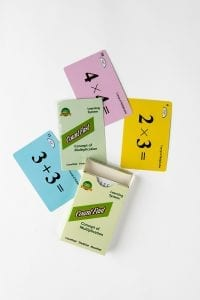# Concept of Multiplication Lesson Plan

####OVERVIEW & PURPOSE

Week 8 of the 2nd Grade CountFast program introduces the concept of multiplication as repeated addition. This module includes some of the basic rules of multiplication with an emphasis on improving fluency and speed with basic multiplication facts. Spend 15 minutes each day on one of the activities listed in this module.  Card decks should go home with students each day for additional practice with a parent at home. Each week, a new deck is introduced and the previous deck is for the student to keep at home for continued practice.

#### EDUCATION STANDARDS

1.  NCTM Standard: understand situations that entail multiplication and division, such as equal groupings of objects and sharing equally.
2.  NCTM Standard: develop fluency in adding and multiplying whole numbers
3.  CCSS.MATH.CONTENT.2.OA.C  Work with equal groups of objects to gain foundations for multiplication.

#### OBJECTIVES

Develop fluency in using the concept of multiplication to quickly solve basic multiplication facts.

#### MATERIALS NEEDED

1.  One CountFast Concept of Multiplication card deck for each student.  This deck is for school and home use.  Discuss routine and expectations for taking home the deck and returning it to school each day.
2.  One writing utensil per student (optional, if you desire students to practice writing the equations)

Concept of Multiplication Day 1

Teacher Model/Direct:  You will use the YELLOW cards from the deck today.  Discuss with students that sometimes we have to add the same number up over and over.  We call this “repeated addition” or “multiplication” (adding the same number a multiple of times).  Show students the rule cards “Zero times any number is Zero” and “One times any number is the number itself”.  Discuss these two rules with the students by using the board to write examples of repeatedly adding up zeros or ones.  Encourage students to remember these two rules for quick mental math with multiplication facts.

Use the rest of the yellow cards to demonstrate that repeated addition and multiplication mean the same thing.  (Two plus two can also be written as two times two – because we are adding the 2 two times.  Notice that the multiplication symbol is simply an addition symbol slightly rotated.)  Skip counting by any number is a way that students have already been exposed to the concept of multiples.  Remind them of skip counting activities they have done in the past and relate that to today’s lesson.  Hold up two related cards (such as 2 + 2 + 2 and 2 X 3) and have students practice saying aloud “Two plus two plus two equals 6, so two times three equals 6. “

Student Activity:  Partner up students and give each pair one deck to work with.  Ask them to take out the yellow cards.  Students should work together to pair up the repeated addition problems with the multiplication problem that means the same thing.  Take turns explaining why each pair has the same answer.  Students should practice verbalizing the concept of multiplication.

Home Activity: Students will take home the deck and the “CountFast Home Connection” letter for this week.  Students will share what they have learned about the concept of multiplication with parents and practice solving each of the yellow cards as quickly as possible.

Concept of Multiplication Days 2 through 5

Teacher Model/Direct:  Repeat the process for the lesson on Day 1, using a different color set from the deck each day.

Day 2 – BLUE cards (multiplying 3)

Day 3 – PINK cards (multiplying 4)

Day 4 – GOLD cards (multiplying 5)

Day 5 – GREEN cards (multiplying 6)

Student Activity:  Partner up students and ask them to take out that day’s color set of cards.  Repeat the student activity from Day 1 with each set.  At the end of the week, student partners can challenge each other by mixing up the entire deck, flipping cards over one at a time, and seeing who can solve each card the fastest.

Home Activity: Students will take home the deck and practice the color set of cards for each day.

#### Instructional Video

Stay SharpFree flash card decks to keep kids entertained and sharp!

To help you keep your kids math sharp during this crisis, we're giving you 3 free CountFast flash card decks for just \$6 in shipping.  Just have them take the quick quiz for their grade at the link below and you'll get a discount code to use on any three decks.